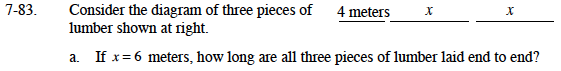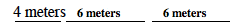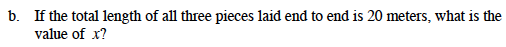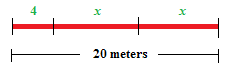### Home > MC1 > Chapter 7 > Lesson 7.2.4 > Problem7-83

7-83.Since x = 6 meters, the three pieces of lumber now look like this:Can you find the total length of the lumber pieces?Using the diagram above, can you write an expression to represent the pieces of lumber?

If 4 + x + x = 20, why must x + x = 16?

Can you find the value of x?